# 模糊逻辑介绍

1 十月 2015, 13:42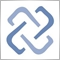1
5 198

### 1. 模糊集合和模糊逻辑的理论背后

• "1" — 如果对象属于一个集合;
• "0" — 如果对象不属一个集合。

### 1.1. 基础特征和定义

1. (30, 0) - 曲线 "年龄 30" 和曲线 "老年" 的交汇点。
2. (30, 0.29) - 曲线 "年龄 30" 和曲线 "中年" 的交汇点。
3. (30, 0.027) - 曲线 "年龄 30" 和曲线 "青年" 的交汇点。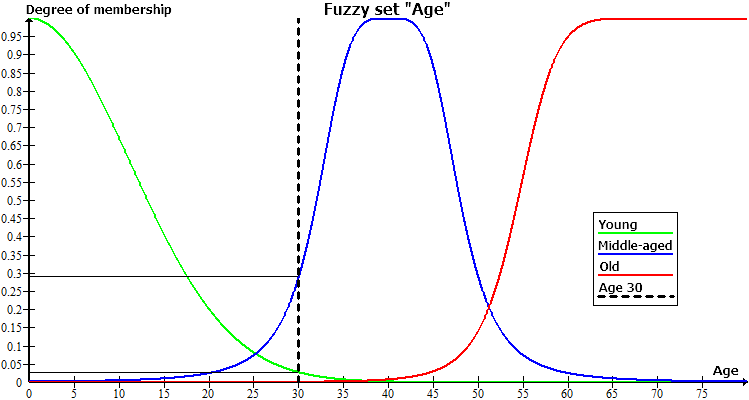### 1.2. 模糊逻辑

1. 定义模型输入和输出特征。
2. 建立一个知识库。
3. 选择模糊推理方法之一。

If 规则条件, then 规则结论。

1. 简单 — 包括一个模糊变量;
2. 复杂 — 包括若干模糊变量.

### 1.3. 马丹尼型模糊推理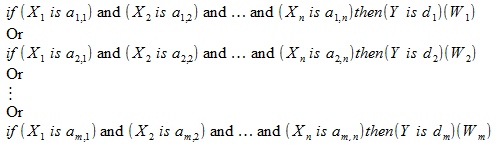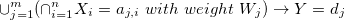•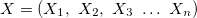— 输入变量向量;
•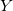输出变量;
•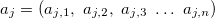输入变量向量 j-th 规则, j = 0,1,2 ... m;
•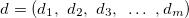— 输出变量的向量值;
•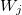权重 j-th 规则, j = 0,1,2 ... m。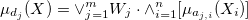— 这个特征函数的工作结果来自知识库的 j-th 规则;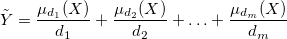1. "截" 隶属函数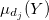于级别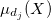;
2. 合并模糊集合。

### 1.4. 关野型模糊推理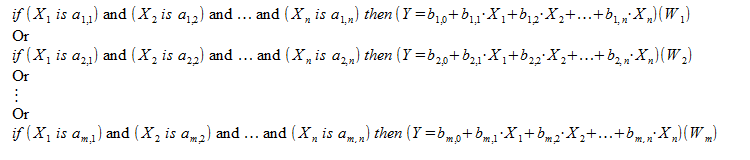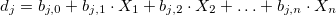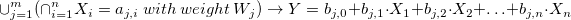•— 输入变量向量
•— 输出变量。
•— 输入变量向量 j-th 规则, j = 0,1,2 ... m。
•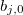— 在用于输出值的线形函数里的自由特征系数 j-th 规则, j = 0,1,2 ... m。
•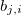i-th 特征的系数, 如果用于输出值的线形函数 j-th 规则, j = 0,1,2 ... m, i = 0,1,2 ... n。
•权重 j-th 规则, j = 0,1,2 ... m。— 这个特征函数的工作结果来自知识库的 j-th 规则;### 2.1. 设计马丹尼型系统

1. 如果 (服务差) 或 (食物垃圾), 则小费吝啬。
2. 如果 (服务好), 则小费一般。
3. 如果 (服务出色) 或 (食物美味), 则小费大方。

1. 我们将会包含文件 MamdaniFuzzySystem.mqh, 它来自 FuzzyNet 的 MQL5 版程序库:
`#include <Math\FuzzyNet\MamdaniFuzzySystem.mqh>`
此文件允许您创建马丹尼型系统, 并操作它们。

2. 现在我们可以创建一个空的马丹尼系统并填充它:
`MamdaniFuzzySystem *fsTips=new MamdaniFuzzySystem();`
3. 我们将创建第一个输入变量 "服务"。当创建模糊变量作为构造器的参数, 首先, 在一行里声明变量名, 随后是它的最小和最大值。
`FuzzyVariable *fvService=new FuzzyVariable("service",0.0,10.0);`
4. 我们为它添加模糊特征。模糊特征构造器使用字符串名字作为第一个参数, 且相应的隶属函数作为第二个参数。
```fvService.Terms().Add(new FuzzyTerm("poor", new TriangularMembershipFunction(-5.0, 0.0, 5.0)));
fvService.Terms().Add(new FuzzyTerm("good", new TriangularMembershipFunction(0.0, 5.0, 10.0)));
fvService.Terms().Add(new FuzzyTerm("excellent", new TriangularMembershipFunction(5.0, 10.0, 15.0)));
```
在此例中所有特征的隶属函数表现为三角函数。

5. 现在已经形成的模糊变量被添加到我们的系统中:
`fsTips.Input().Add(fvService);`
6. 与此类似, 我们实现了第二个输入变量 "food", 但此变量特征将拥有梯形隶属函数。
```FuzzyVariable *fvFood=new FuzzyVariable("food",0.0,10.0);
fvFood.Terms().Add(new FuzzyTerm("rancid", new TrapezoidMembershipFunction(0.0, 0.0, 1.0, 3.0)));
fvFood.Terms().Add(new FuzzyTerm("delicious", new TrapezoidMembershipFunction(7.0, 9.0, 10.0, 10.0)));
```
7. 由于该系统具有马丹尼推理, 其输入和输出值用相同的方法来确定。因此, 以相同的方式, 我们创建了类似的输出:
```FuzzyVariable *fvTips=new FuzzyVariable("tips",0.0,30.0);
fvTips.Terms().Add(new FuzzyTerm("cheap", new TriangularMembershipFunction(0.0, 5.0, 10.0)));
fvTips.Terms().Add(new FuzzyTerm("average", new TriangularMembershipFunction(10.0, 15.0, 20.0)));
fvTips.Terms().Add(new FuzzyTerm("generous", new TriangularMembershipFunction(20.0, 25.0, 30.0)));
```
8. 创建模糊规则, 它们一起作为我们的系统知识库。为创建一条规则, 它需要请求调用来自对象的方法 ParseRule, 表示我们的系统, 且作为一个选项, 给模糊规则一个简单的字符串表示:
```MamdaniFuzzyRule *rule1 = fsTips.ParseRule("if (service is poor) or (food is rancid) then (tips is cheap)");
MamdaniFuzzyRule *rule2 = fsTips.ParseRule("if (service is good) then (tips is average)");
MamdaniFuzzyRule *rule3 = fsTips.ParseRule("if (service is excellent) or (food is delicious) then (tips is generous)");
```

编写模糊规则要求严格输入, 并且不允许使用非关键词。 关键词是: "if", "then", "is", "and", "or", "not", "(", ")", "slightly", "somewhat", "very", "extremely "。最后四个关键词是语义量词。此外, 在您的系统上可用的所有变量名, 特征和函数都包含在关键字列表中。模糊特征的重要性或关野线形函数是递增的, 或与之相反, 语义量词递减。以下实现的语义量词适用:

1. "slightly" — 以立方根替代条件结果。极大降低数值。
2. "somewhat" — 以平方根替代条件结果。降低数值。
3. "very" — 将条件结果带到第二级。增加数值。
4. "extremely" — 将条件结果带到第三级。极大增加数值。

9. 剩下的步骤是在系统里添加规则:
```fsTips.Rules().Add(rule1);
```

### 2.2. 设计关野型系统

• 车辆当前时速, 与巡航控制系统中设置的速度差值;
• 差值降低 (增加) 有多迅速。

1. :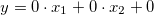;
2. 较快: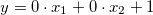;
3. 较慢: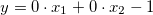;
4. 函数: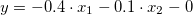.

1. If (速度误差较慢), and (速度误差点较慢), 加速较快。
2. If (速度误差较慢), and (速度误差点为零), 加速较快。
3. If (速度误差较慢), and (速度误差点较快), 加速为零。
4. If (速度误差为零), and (速度误差点较慢), 加速较快。
5. If (速度误差为零), and (速度误差点为零), 加速为函数。
6. If (速度误差为零), and (速度误差点较快), 加速较慢。
7. If (速度误差较快), and (速度误差点较慢), 加速较快。
8. If (速度误差较快), and (速度误差点为零), 加速较慢。
9. If (速度误差较快), and (速度误差点较快), 加速较慢。

1. 我们包含文件 SugenoFuzzySystem.mqh, 它来自 FuzzyNet 的 MQL5 版程序库:
`#include <Math\FuzzyNet\SugenoFuzzySystem.mqh>`
此文件允许您创建关野型系统, 并操作它们。

2. 现在我们可以创建一个空的关野系统并填充它:
`SugenoFuzzySystem *fsCruiseControl=new SugenoFuzzySystem();`
3. 关野系统的输入变量创建过程与马丹尼型系统一样。

我们将创建变量 "速度误差" 并将之添加到系统:

```FuzzyVariable *fvSpeedError=new FuzzyVariable("SpeedError",-20.0,20.0);
fvSpeedError.Terms().Add(new FuzzyTerm("zero", new TriangularMembershipFunction(-15.0, -0.0, 15.0)));
fvSpeedError.Terms().Add(new FuzzyTerm("faster", new TriangularMembershipFunction(5.0, 20.0, 35.0)));
```

我们将创建变量 "SpeedErrorDot" 并将之添加到系统:

```FuzzyVariable *fvSpeedErrorDot=new FuzzyVariable("SpeedErrorDot",-5.0,5.0);
fvSpeedErrorDot.Terms().Add(new FuzzyTerm("slower", new TriangularMembershipFunction(-9.0, -5.0, -1.0)));
fvSpeedErrorDot.Terms().Add(new FuzzyTerm("zero", new TriangularMembershipFunction(-4.0, -0.0, 4.0)));
fvSpeedErrorDot.Terms().Add(new FuzzyTerm("faster", new TriangularMembershipFunction(1.0, 5.0, 9.0)));
```
4. 我们将创建关野型模糊变量, 这将是该系统的输出。当创建模糊变量时, 构造器仅接受它的名字作为参数。可为它添加线形函数, 但首先, 这些函数需要确定, 且我们需要一个 双精度 型的系数数组。

线形函数 的形成是按以下方式进行: 每个输入变量是一个等式的未知数, 系数取自一个系数数组。这种方式, 在数组中的系数必须按照输入变量的相同记录顺序放置。因此, 对于第一个输入变量, 系数的下标应为 0, 对于第二个 - 下标为 1, 等等。所以, 系数数组的长度必须大于一个单元, 或等于输入变量的数量。如果长度相等, 则自由特征的系数等于零, 否则其值将等于数组最后一个元素。

在我们的系统中有两个输入变量, 所以系数数组的长度应不超过 3。我们将声明所有的四个数组, 根据它们的信息, 我们将按照上述描述形成函数, 并将它们添加到关野型模糊变量, 然后将它们包括在系统中:
```SugenoVariable *svAccelerate=new SugenoVariable("Accelerate");
double coeff1={0.0,0.0,0.0};
double coeff2={0.0,0.0,1.0};
double coeff3={0.0,0.0,-1.0};
double coeff4={-0.04,-0.1,0.0};
```
5. 有别于马丹尼系统, 我们将创建九条规则:
```SugenoFuzzyRule *rule1 = fsCruiseControl.ParseRule("if (SpeedError is slower) and (SpeedErrorDot is slower) then (Accelerate is faster)");
SugenoFuzzyRule *rule2 = fsCruiseControl.ParseRule("if (SpeedError is slower) and (SpeedErrorDot is zero) then (Accelerate is faster)");
SugenoFuzzyRule *rule3 = fsCruiseControl.ParseRule("if (SpeedError is slower) and (SpeedErrorDot is faster) then (Accelerate is zero)");
SugenoFuzzyRule *rule4 = fsCruiseControl.ParseRule("if (SpeedError is zero) and (SpeedErrorDot is slower) then (Accelerate is faster)");
SugenoFuzzyRule *rule5 = fsCruiseControl.ParseRule("if (SpeedError is zero) and (SpeedErrorDot is zero) then (Accelerate is func)");
SugenoFuzzyRule *rule6 = fsCruiseControl.ParseRule("if (SpeedError is zero) and (SpeedErrorDot is faster) then (Accelerate is slower)");
SugenoFuzzyRule *rule7 = fsCruiseControl.ParseRule("if (SpeedError is faster) and (SpeedErrorDot is slower) then (Accelerate is faster)");
SugenoFuzzyRule *rule8 = fsCruiseControl.ParseRule("if (SpeedError is faster) and (SpeedErrorDot is zero) then (Accelerate is slower)");
SugenoFuzzyRule *rule9 = fsCruiseControl.ParseRule("if (SpeedError is faster) and (SpeedErrorDot is faster) then (Accelerate is slower)");
```
6. 我们将它们添加到我们的系统:
```fsCruiseControl.Rules().Add(rule1);
```

### 2.3. 马丹尼型和关野型系统的计算

```CList *in=new CList;
Dictionary_Obj_Double *p_od_Service=new Dictionary_Obj_Double;
Dictionary_Obj_Double *p_od_Food=new Dictionary_Obj_Double;
p_od_Service.SetAll(fvService, Service);
p_od_Food.SetAll(fvFood, Food);
```

```CList *result=new CList;
Dictionary_Obj_Double *p_od_Tips=new Dictionary_Obj_Double;
```

```result=fsTips.Calculate(in);
p_od_Tips=result.GetNodeAtIndex(0);
```

```CList *in=new CList;
Dictionary_Obj_Double *p_od_Error=new Dictionary_Obj_Double;
Dictionary_Obj_Double *p_od_ErrorDot=new Dictionary_Obj_Double;
p_od_Error.SetAll(fvSpeedError,Speed_Error);
p_od_ErrorDot.SetAll(fvSpeedErrorDot,Speed_ErrorDot);
CList *result=new CList;
Dictionary_Obj_Double *p_od_Accelerate=new Dictionary_Obj_Double;
result=fsCruiseControl.Calculate(in);
p_od_Accelerate=result.GetNodeAtIndex(0);
```

### 参考和链接的清单| 16 11月 2015 在 08:23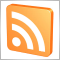在MetaTrader5中创建交互应用来展现RSS订阅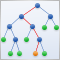用随机森林预测趋势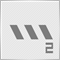在 MetaTrader 5 里使用 HedgeTerminal (对冲终端) 面板进行双向交易和仓位对冲, 第二部分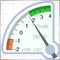使用 CCanvas 类绘制刻度表盘